CLAT  >  Test: Problems On Ages - 1

# Test: Problems On Ages - 1

Test Description

## 10 Questions MCQ Test Quantitative Techniques for CLAT | Test: Problems On Ages - 1

Test: Problems On Ages - 1 for CLAT 2023 is part of Quantitative Techniques for CLAT preparation. The Test: Problems On Ages - 1 questions and answers have been prepared according to the CLAT exam syllabus.The Test: Problems On Ages - 1 MCQs are made for CLAT 2023 Exam. Find important definitions, questions, notes, meanings, examples, exercises, MCQs and online tests for Test: Problems On Ages - 1 below.
Solutions of Test: Problems On Ages - 1 questions in English are available as part of our Quantitative Techniques for CLAT for CLAT & Test: Problems On Ages - 1 solutions in Hindi for Quantitative Techniques for CLAT course. Download more important topics, notes, lectures and mock test series for CLAT Exam by signing up for free. Attempt Test: Problems On Ages - 1 | 10 questions in 20 minutes | Mock test for CLAT preparation | Free important questions MCQ to study Quantitative Techniques for CLAT for CLAT Exam | Download free PDF with solutions
 1 Crore+ students have signed up on EduRev. Have you?
Test: Problems On Ages - 1 - Question 1

### Ten years ago, P was half of Q's age. If the ratio of their present ages is 3:4, what will be the total of their present ages?

Detailed Solution for Test: Problems On Ages - 1 - Question 1

Let present age of P and Q be 3x
and  4x  respectively.
Ten years ago, P was half of Q's age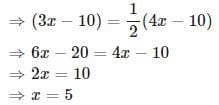Total of their present ages
=3x+4x=7x=7×5=35

Test: Problems On Ages - 1 - Question 2

### Father is aged three times more than his son Sunil. After 8 years, he would be two and a half times of Sunil's age. After further 8 years, how many times would he be of Sunil's age?

Detailed Solution for Test: Problems On Ages - 1 - Question 2

Assume that Sunil's present age
= x.
Then, father's present age  =3x + x = 4x

After 8 years, father's age =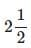times of Sunils' age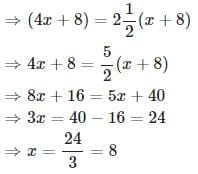After further  8  years,
Sunil's age  = x + 8 + 8 = 8 + 8 + 8 = 24
Father's age  = 4 x + 8 + 8 = 4 x 8 + 8 + 8 = 48

Father's age/Sunil's age = 48/24 = 2

Test: Problems On Ages - 1 - Question 3

### A man's age is 125%of what it was 10 years ago, but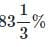of what it will be after 10years. What is his present age?

Detailed Solution for Test: Problems On Ages - 1 - Question 3

Let the age before 10 years =x. Then,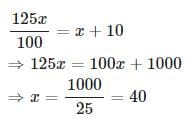Present age = x+10=40+10=50

Test: Problems On Ages - 1 - Question 4

A man is 24 years older than his son. In two years, his age will be twice the age of his son. What is the present age of his son?

Detailed Solution for Test: Problems On Ages - 1 - Question 4

Let present age of the son = x years
Then, present age the man = ( x + 24 ) years

Given that, in 2 years, man's age will be twice the age of his son
⇒(x+24)+2=2(x+2)
⇒x=22

Test: Problems On Ages - 1 - Question 5

Present ages of Kiran and Syam are in the ratio of 5 : 4 respectively. Three years hence, the ratio of their ages will become 11 : 9 respectively. What is Syam's present age in years?

Detailed Solution for Test: Problems On Ages - 1 - Question 5

Ratio of the present age of Kiran and Syam = 5 : 4

Let present age of Kiran = 5x
Present age of Syam = 4x
After 3 years, ratio of their ages = 11 : 9
⇒ ( 5 x + 3 ) : ( 4 x + 3 ) = 11 : 9
⇒ 9 ( 5 x + 3 ) = 11 ( 4 x + 3 )
⇒ 45 x + 27 = 44 x + 33
⇒ x = 33 − 27 = 6
Syam's present age = 4 x = 4 × 6 = 24

Test: Problems On Ages - 1 - Question 6

The sum of ages of 5 children born at the intervals of 3 years each is 50 years. Find out the age of the youngest child?

Detailed Solution for Test: Problems On Ages - 1 - Question 6

Let the age of the youngest child = x
Then, the ages of 5 children can be written as x , ( x + 3 ) , ( x + 6 ) , ( x + 9 ) and ( x + 12 )
x + ( x + 3 ) + ( x + 6 ) + ( x + 9 ) + ( x + 12 ) = 50
⇒ 5 x + 30 = 50
⇒ 5 x = 20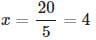Test: Problems On Ages - 1 - Question 7

A is two years older than B who is twice as old as C. The total of the ages of A, B and C is 27 . How old is B?

Detailed Solution for Test: Problems On Ages - 1 - Question 7

Let age of C = x .

Then, Age of B = 2x
Age of A = 2 + 2x
Total age of A,B and C =27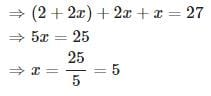B's age =2x=2×5=10

Test: Problems On Ages - 1 - Question 8

The average age of a class of 22 students is 21 years. The average increased by 1 when the teacher's age also included. What is the age of the teacher?

Detailed Solution for Test: Problems On Ages - 1 - Question 8

Total age of all students
= 22 × 21

Total age of all students + Age of the teacher  = 23 × 22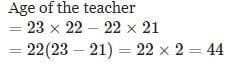Test: Problems On Ages - 1 - Question 9

A father said to his son, "I was as old as you are at the present at the time of your birth". If the father's age is 38 years now, what was the son's age five years back?

Detailed Solution for Test: Problems On Ages - 1 - Question 9

Let son's present age be x years. Then,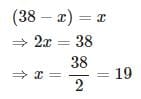Son's age 5  years back = 19 − 5 = 14

Test: Problems On Ages - 1 - Question 10

Ayisha's age is 1 / 6 th of her father's age. Ayisha's father's age will be twice Shankar's age after 10 years. If Shankar's eight birthdays was celebrated two years before, then what is Ayisha's present age.

Detailed Solution for Test: Problems On Ages - 1 - Question 10

Let Ayisha's present age = x
Then, her father's age = 6 x
Given that Ayisha's father's age will be twice Shankar's age after 10 years. Therefore, Shankar's age after 10 years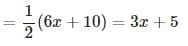Also given that Shankar's eight birthdays was celebrated two years before. Therefore, Shankar's age after 10 years = 8 + 12 = 20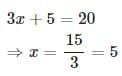Therefore, Ayisha's present age =5 years

## Quantitative Techniques for CLAT

56 videos|35 docs|91 tests
 Use Code STAYHOME200 and get INR 200 additional OFF Use Coupon Code
Information about Test: Problems On Ages - 1 Page
In this test you can find the Exam questions for Test: Problems On Ages - 1 solved & explained in the simplest way possible. Besides giving Questions and answers for Test: Problems On Ages - 1, EduRev gives you an ample number of Online tests for practice

## Quantitative Techniques for CLAT

56 videos|35 docs|91 tests